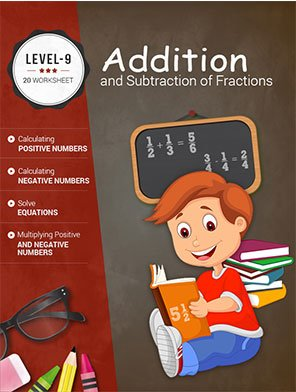\$5.00

## LEVEL 9 WORKSHEETS

Level 9 starts with number concept. Students will learn reducing fractions, addition, subtraction and multiplication of fractions and mixed numbers. The students will get the knowledge of statistics.

Worksheet # Topics/Curriculum # of Pages 2pg Sample Add to cart
Unit-1 Number Concepts 10 pages\$1.00

Unit-2 Introducing Fractions 10 pages\$1.00

Unit-3 Reducing Fractions Using GCF 10 pages\$1.00

Unit-4 More Problems on Addition of Unlike Fractions 10 pages\$1.00

Unit-5 Addition of Unlike Fractions and Mixed Numbers 10 pages\$1.00

Unit-6 Subtraction of Fractions, Mixed Numbers, and Whole Numbers 10 pages\$1.00

Unit-7 Subtraction of Unlike Fractions and Mixed Numbers 10 pages\$1.00

Unit-8 Addition and Subtraction of Fractions and Mixed Numbers 10 pages\$1.00

Unit-9 Multiplication of Fractions and Mixed Numbers 10 pages\$1.00

Unit-10 More Problems on Multiplication of Fractions and Mixed Numbers 10 pages\$1.00

Unit-11 Division of Fractions and Mixed Numbers 10 pages\$1.00

Unit-12 Multiplication and Division of Fractions and Mixed Numbers 10 pages\$1.00

Unit-13 Adding of Three Fractions 10 pages\$1.00

Unit-14 Multiplication of Decimals 10 pages\$1.00

Unit-15 Division of Decimals 10 pages\$1.00

Unit-16 Introducing Integers (Z) 10 pages\$1.00

Unit-17 Adding and Subtracting Positive and Negative Numbers 10 pages\$1.00

Unit-18 Calculating Positive and Negative Numbers 10 pages\$1.00

Unit-19 Multiplying Positive and Negative Numbers 10 pages\$1.00

Unit-20 Dividing Positive and Negative Numbers 10 pages\$1.00

Unit-21 Simplifying Positive and Negative Numbers 10 pages\$1.00

Unit-22 Finding Values in Equations 10 pages\$1.00

Unit-23 Solving Equations 10 pages\$1.00

Unit-24 More Problems on Solving Equations 10 pages\$1.00

Unit-25 Solving Equations with Parentheses 10 pages\$1.00

Unit-26 Ratio, Percent, and Proportion 10 pages\$1.00

Unit-27 Practical Arithmetic 10 pages\$1.00

Unit-28 Two-Dimensional Figures 10 pages\$1.00

Unit-29 Three-Dimensional Measurement Formulae 10 pages\$1.00

Unit-30 Introduction to Statistics 10 pages\$1.00